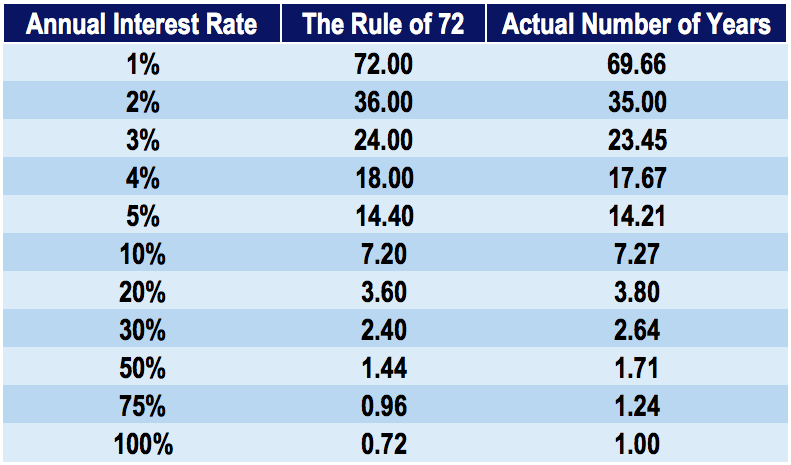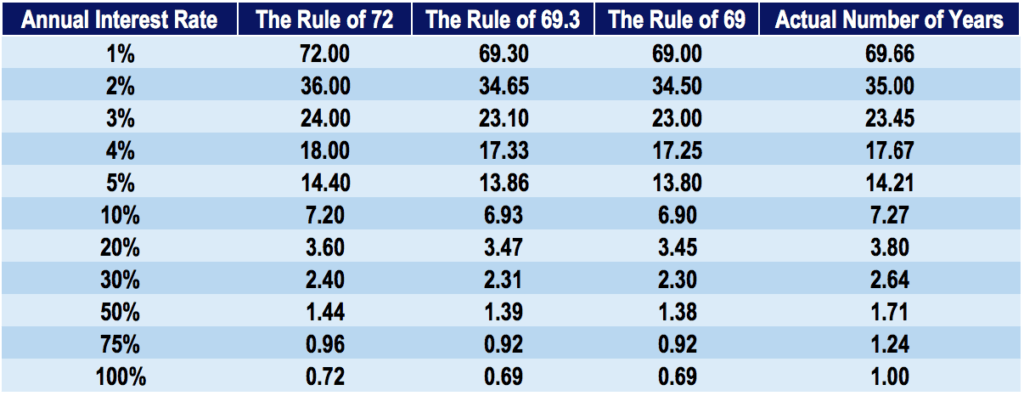# Rule of 72

The length of time required for an investment to double in value at a fixed annual rate of return

## What is the Rule of 72?

In finance, the Rule of 72 is a formula that estimates the amount of time it takes for an investment to double in value, earning a fixed annual rate of return. The rule is a shortcut, or back-of-the-envelope, calculation to determine the amount of time for an investment to double in value. The simple calculation is dividing 72 by the annual interest rate.

### Time (Years) to Double an Investment

The Rule of 72 gives an estimation of the doubling time for an investment. It is a fairly accurate measurement, and more so when using lower interest rates rather than higher ones. It is used for situations involving compound interest. A simple interest rate does not work very well with the Rule of 72. Below is a table showing the difference between the Rule of 72 calculation and the actual number of years required for an investment to double in value:### Rule of 72 Formula

The Rule of 72 formula is as follows:### Example of the Rule of 72

You are the owner of a coffee machine manufacturing company. Due to the large capital needed to establish a factory and warehouse for coffee machines, you have turned to private investors to fund the expenditure. You meet with John, who is a high net-worth individual willing to contribute \$1,000,000 to your company. However, John is only willing to contribute said amount on the presumption that he will get a 12% annual rate of return on his investment, compounded yearly. He wants to know how long it will take for his investment in your company to double in value.

Using the Rule of 72:It will take approximately six years for John’s investment to double in value.

### Deriving the Rule of 72

Let us derive the Rule of 72 by starting with a beginning arbitrary value: \$1. Our goal is to determine how long it will take for our money (\$1) to double at a certain interest rate.

Suppose we have a yearly interest rate of “r”. After one year, we will get:

\$1 x (1+r)

At the end of two years, we will get:

\$1 x (1+r) x (1+r)

Extending this year after year, we get:

\$1 x (1+r)^n, where n = number of years

If we want to determine how long it takes to double our money, turning \$1 into \$2:

\$1 x (1+r)^n = \$2

Solving for years (n):

Step 1: \$1 x (1+r)^n = \$2

Step 2: (1+r)^n = \$2

Step 3: ln((1+R)^n) = ln(2)              (Taking the natural log of both sides)

Step 4: n x ln(1+r) = .693

Step 5: n x r = 0.693                       (Approximation that ln(1+r) = r)

Step 6: n = .693 / r

Step 7: n = 69.3 / r                         (Turning r into an integer rather than a decimal)

Notice that after deriving the formula, we end up with 69.3, not 72. Although 69.3 is more accurate, it is not easily divisible. Therefore, the Rule of 72 is used for the sake of simplicity. The number 72 also provides more factors (2, 3, 4, 6, 12, 24…).

### Rules of 72, 69.3, and 69

Rules of 69.3 and of 69 are also methods of estimating an investment’s doubling time. The rule of 69.3 is considered more accurate than the Rule of 72, but can be much more troublesome to calculate. Therefore, investors typically prefer to use a rule of 69 or 72 rather than the rule of 69.3.

Comparing the doubling time for rules of 69, 69.3, and 72 to actual years:As you can see from the table above, the rule of 69.3 yields more accurate results at lower interest rates. However, as the interest rate increases, the rule of 69.3 loses some of its predictive accuracy.

The Rule of 72 is a simple, helpful tool that investors can use to estimate how long a specific compound interest investment will take to double their money.

### More Resources

Thank you for reading CFI’s explanation of the Rule of 72. Our mission is to help you advance your career. Below are additional free resources from CFI, the global provider of the Financial Modeling & Valuation Analyst (FMVA) certification program:

• Investing: A Beginner’s Guide
• Hurdle Rate
• Return on Investment (ROI) Formula
• Financial Modeling Courses Collection

### Corporate Finance Training

Advance your career in investment banking, private equity, FP&A, treasury, corporate development and other areas of corporate finance.

Enroll in CFI’s Finance Courses to take your career to the next level! Learn step-by-step from professional Wall Street instructors today.